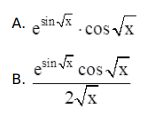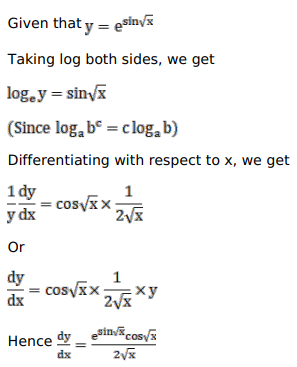# Solve this following

Question:

Mark $(\sqrt{ })$ against the correct answer in the following:

If $y=e^{\sin \sqrt{x}}$ then $\frac{d y}{d x}=?$C. $\frac{e^{\sin \sqrt{x}}}{2 \sqrt{x}}$

D. none of these

Solution: